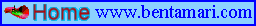﻿ Attractors

Attractors

Published in 2000; updated on October 14, 2019.

••••••••••••••••••••••••••••••••••••••••••••••••••••••••••••••••••••••••••••••••••••••••••••••••••••••••••••••••••••••••••••••••••••••••••••••••••••••••••••••••••••••••••••••••••••••
Please note: Best viewed at 1280 x 1024 resolution.
Texts in blue italics are "
quotes."
Texts in blue underline are links.
Texts with
N signify new or updated text.
Texts in black on white background are my texts, including My Fragments (MF#).
You are allowed to copy from my texts or pictures, providing the copyright is properly attributed to: ©Ben Tamari www.bentamari.com.
••••••••••••••••••••••••••••••••••••••••••••••••••••••••••••••••••••••••••••••••••••••••••••••••••••••••••••••••••••••••••••••••••••••••••••••••••••••••••••••••••••••••••••••••••••••

Attractor Quotes

Peitgen, H.O., Jurgens, H. and Saupe, D. (1992). "Chaos and Fractals, New Frontiers of Science." Springer-Verlag. p. 656.

"Strange attractors are the point where chaos and fractals meet in an unavoidable and most natural fashion:
As geometrical patterns, strange attractors are fractals; as dynamical objects, strange attractors are chaotic.
"

Bak, P. (1997). "How Nature Works: The science of self-organized criticality." Cambridge University Press, p. 31.

"Strange attractors have fractal properties, but they do not represent geometrical fractals in real space like those we see in nature."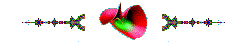My Fragments - From my personal point of view

For the complete list, please see Tamari.

MF1:  Fractals relate to attractors like cobblestones in roads.   Added 1 January 2000.Attractors, on this page, are mathematical entities: models, tools, machines and engines, for handling natural phenomena such as climate, chemistry and economics.

1D - One-dimensional attractors:

Logistic Equation - Cobweb model - Populations

x' = ax (1-x)

a = 3.92D - Two-dimensional attractors:

Double Pendulum

x1 = l1sinθ1
y1 = -l1cosθ1
x2 = l1sinθ1 + l2sinθ2
y2 = -l1cosθ1 - l2cosθ2

http://en.wikipedia.org/wiki/Double pendulum

Duffing Attractor

x' = y
y' = x - x3 - ay + bcos(ct)

a = 0.25,   b = 0.3,   c = 1

x0 , y0 , z0 = 0,     -2 ≤ x , y ≤ 2

Henon Attractorx' = 1 - ax2 + y
y' = bx

a = 1.4 ,  b= 0.3

x0 ,y0 = 1 ,    -1.5 ≤ x , y ≤ 1.5

Swinging Atwood's machinex'' ≡ r'' = (rɵ2 + g (cosɵ-µ)) / (1+µ)
y' ≡ ɵ'' = - [(2r'ɵ' + g sinɵ) / (r)]
g is the gravitational acceleration
µ is the ratio between the two masses (µ = M/m =2).
Source: http://demonstrations.wolfram.com/SwingingAtwoodsMachine/

Tinkerbell Attractorx' = x2 - y2< + ax + by
y' = 2xy - cx + dy

a = 0.9 ,  b = -0.6 ,   c = 2 , d = 0.5

KAM Torus  Kolmogorov Arnold Moserx' = xcos(ζ) + (x2 - y)sin(ζ)
y' = xsin(ζ) - (x22 - y)cos(ζ)

x' = sin(ay) - cos(bx)
y' = sin(cx) - cos(dy )3D - Three-dimensional attractors: Chua, Ikeda, Lorenz, Pickover, Rossler, and Tamari attractors, figured with Eco software.

Chua Attractor - Electric circuitsx' = a(y - x - g)
y' = x - y + z
z' = by - cz

g = ex + (d-e)[abs(x+1)-abs(x-1)]/2 ,   a = 15.6 ,   b = 28 ,   c = 0 ,   d = -1.143 ,   e = -0.714

x0 = 0.7 ,   y0 = 0 ,   z0 = 0 ,       -2 ≤ x,≤  2, 3 ≤y,z,≤3

Ikeda Attractor - Laser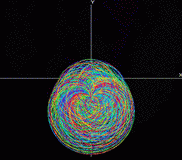x' = a + b(xcosz - ysinz )
y' = b (xsinz + ycosz)
z' = c - d / (1+x2 + y2)

a = 1 ,   b = 0.9 ,   c = 0.4 ,   d = 6

x0, y0, z0 = 0 ,   - 2 ≤ x, y ≤ 2

Lorenz Attractor - Meteorology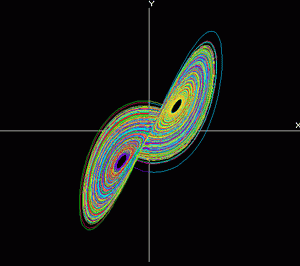x' = x - (ax + ay)           "x" convective flow
y' = y + (bx - y - zx)     "y" horizontal temperature distribution
z' = z - (cz + xy)            "zvertical temperature distribution

a = 28 ,   b = 8/3 .   c= 10

x0 = 0 ,   y0 = 1 ,   z0 = 0 ,       -1 ≤ x, y, z, ≤ 2

Pickover Attractor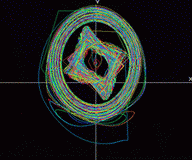x' = sin(ax) - zcos(by)
y' = zsin(cx) - cos(dy)
z' = e / sin(x)

a = 2.24 ,   b = 0.43 ,  c = - 0.65 ,  d = - 2.43 ,  e = 1

x0, y0, z0 = 0 ,     - 2 ≤ x, y ≤ 2

Rossler Attractor - Chemistryx' = x - (y - x)
y' = y + (x + ay)
z' = z + (b + xz - cz)

a = 0.2 ,   b = 0.2 ,   c= 5.7

x0 = 1 ,   y0 = 0 ,   z0 = 0 ,          -1 ≤ x , y, z, ≤ 1

Tamari Attractor - Economic Attractor - Economics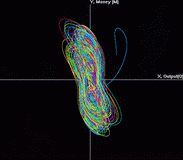variables:
x' = (x - ay)cos(z) - bysin(z)                       "x" output
y' = (x + cy)sin(z) + dycos(z)                      "ymoney
z' = e + fz + gatan{ [(1 - u)y] / [(1 - i)x] }   "z" pricing - spiral version
z' = e + fz + gatan{ (1 - u) / (1 - i) xy }       "z" wealth - attractor version

Exogens:
aInertia = 1.013
bProductivity = - 0.011
cPrinting = 0.02
dAdaptation= 0.96
eExchange = 0
fIndexation = 0.01
gElasticity/Expectations= 1
uUnemployment = 0.05
iInterest= 0.05

x0, y0, z0, = 1 ,   1 ≤ x, y, ≤ 4

Source: Tamari, B. (1997). "Conservation and Symmetry Laws and Stabilization Programs in Economics."

Economic Equilibrium Points in time: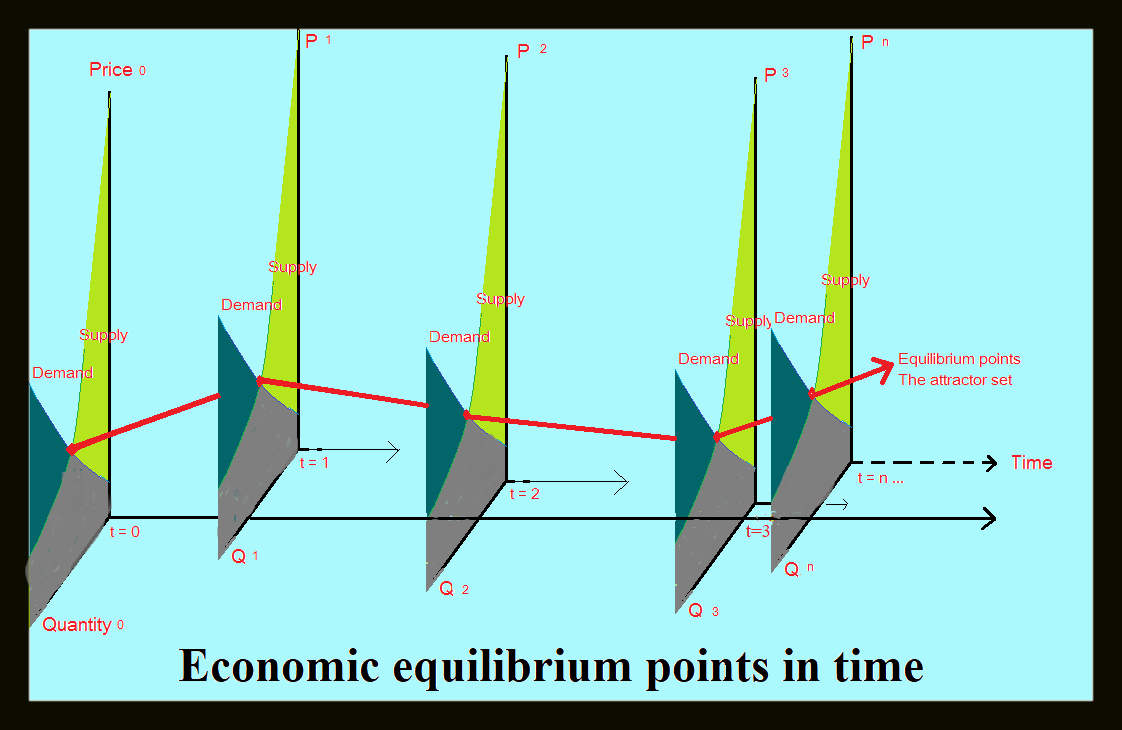Zeleny, E. "Tamari Attractor." From the Wolfram Demonstrations Project.

"Tamari attractor".

Abstract: This bachelor thesis deals with the Tamari attractor problem and its application for forming a prediction model. The core of the work is to create a simulation program in the MATLAB development environment and to use it to create and compare several case studies of a predictive model based on different parameters. This model is graphically illustrated and supplemented by economic interpretation.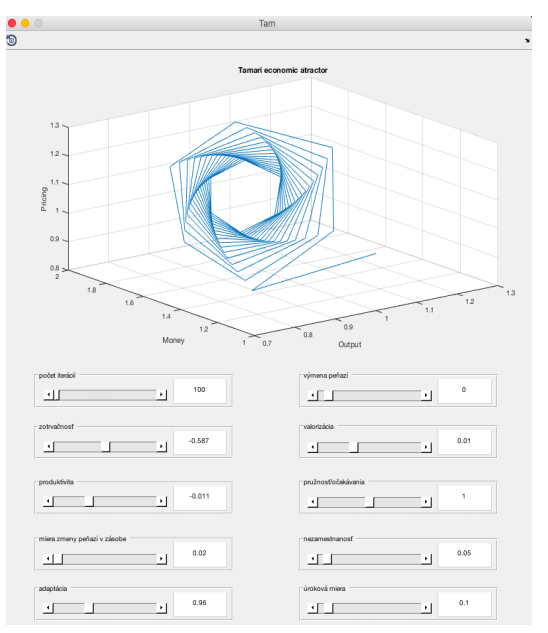Introductory Tamari Attractor At Startup

http://www.deviantart.com/art/Tamari-Attractor-120420405  Tamari attractor."Tamari Attractor" Formula is a modification of the Tamari Attractor.Note 1, 12 August 2006   Note on Tamari space - the "Nest":

Sprott, J. C.  (1993) "Strange Attractors: Creating Patterns in Chaos." M&T Books, NY.

"To assess how common chaos is in nature, we must address the more complicated and subjective issue of whether the equations we have examined are a representative sample of the equations that describe natural processes. ... Chaos is not the most common behavior, but it is neither particularly rare."

The origin of the Nest is the Tamari space on which the economic system, i.e. the Tamari attractor, sails.

O' = (O - aM)cos(P) - bMsin(P)                         "O" Output
M' = (O + cM)sin(P) + dMcos(P)                       "M" Money
P' = e + fP + garctan{[(1-u)M] / [(1-i)O]}          "P" Pricing

(1-u) ≡ Gresham coefficient     (1-u)M represents demand
(1-i)  ≡ Gresham coefficient      (1-i)O represents supply

The Nest is the economic surface on which economic systems sail (in the deterministic sense) and wander (in the random sense). In a particular range of parameters, the Nest constitutes an attractor.

Some scientists have scientific problems and look for tools (mathematical, statistical or graphic) to solve them, whereas some scientists have tools and look for scientific problems on which to use them. This is identical to a person who has a nail and is looking for a hammer or, alternatively, has a hammer and is looking for a nail. Anyone dealing with a scientific field, e.g., biology, chemistry, economy, physics, psychology ('science-fiction' and 'speculations' are now also considered legitimate sciences), finds himself in one of these two positions.

When I was working as an economist in Israel's Economic Planning Authority in the 70s, we were asked to perform annual forecasts and prepare five-year economic plans. The planning and the forecasting were usually conducted using the naive (inertia) method, according to which one took the last growth rates of the various parameters and projected (extrapolated) them to the future. This worked as long as no inflation existed and the country was growing economically. That is, the inertia was enough for the forecasting and planning.

With the change of the ruling party in Israel in 1977, the economical navigation policy changed as well. From a social-democratic society (characterized by solidarity and planning), we have turned, slowly but surely, into a capitalist-liberal state (Darwinian and competitive). Inflation raised its head, forecasting and planning became more difficult and problematic, were left out, and then disappeared from public-economic thinking and navigation processes. The various government think tanks were closed one by one, and even the Economic Planning Authority in the Prime Minister's office was dismantled.

The planning procedure at that time involved using econometric models for forecasting and planning. These, together with computerized spreadsheets, gave us extensive computing ability, but little mathematical capacity or knowledge (in the Sprott sense, quoted above).

As one who believes that 'economics is physics with pricing' or 'physics is economics without pricing' (how nature prices itself, and what kind of money it uses, is not relevant to this remark), it was clear to me that what was lacking for proper planning and forecasting processes were economy 'behavior equations.' My first attempt to add an appropriate mathematical tool to the system (shelf solution) is described in the article "Prices and Quantities in the Israeli Housing Market" (1981), the main idea of which was using the mechanism of cobwebs to describe the behavior of the housing market in Israel.

With the rise of inflation it seemed that the most important variable would be the quantity of money (M) which had a crucial influence on the rest of the variables in the system. However, the expected correlation between money (M) and pricing (P) surprisingly could not be found, and that in itself required an explanation.

I therefore showed that the mechanism of money transfer into the market is not direct but involves the impact of "Gresham's Law" (1983). In those days, the accepted way to deal with the influence of money on the economy was with what is called the "real balances effect" (M/P), and it was common to assume that as long as the economic variables were linked to the Consumer Price Index, inflation would be neutral.

In actually, a mechanic feedback process was in effect, feeding itself and creating a "perpetuum mobile." The printing of money accelerates inflation and that, through the linked mechanisms, accelerates money-printing. Very quickly it was discovered that real balances are in fact a double-edged sword. When they exceed a certain critical amount, their tendency reverses. The public does not accumulate them anymore but actually withdraws from them, and this accelerates inflation.

I demonstrated this idea in the article "Anatomy of a Tragedy" (1985) by showing that the scale itself doesn't really matter; be it a large country or a small one, the same rule applies (i.e., what we today call a fractal structure or universality). The conclusion was that the important variable is not real balances, but the amount of money relative to output (M/O). This idea was further developed in my article "Theoretical and Empirical Inflation" (1986).

The main question in my work is what happens when money (M) is introduced as a variable in utility and production functions, and an especially important question is what happens in an economic system when the amount of money is raised indefinitely. It quickly turns out that the real issues of debate are ones of measurer and measured, whereas the measurer (M) is a part of a measured system [Ot, Mt, t; Output, Money, time].

As an economist by training, and not a mathematician, I was looking for a mathematical shelf-solution in order to progress. At first, I worked only in an output-money space [Ot, Mt, t; Output, Money, time]. In the article "Why Is There No Growth in Israel?" (1990). I described how a surplus of money curved the economic space and caused the economic units to deviate from their optimal conditions. Since I found that an economic system in the output and money space is a conservative one, I chose Cremona equations (which have a conservative quality in a two-dimensional space) as being suitable for depicting the output-money [Ot, Mt, t; Output, Money, time] space.

The real equations, in the sense of the quote given above, are most likely modifications of Cremona equations. It is also likely that the econometric findings produced by the real equations are not supposed to change on principle, especially not the sign of the money parameter (- b) in the first equation. I explained these ideas in the book Ecometry - Foundations of Economics (1991, Hebrew). The name Ecometry is derived from the combinations of the words Eco-nomy and Geo-metry.

The basic hypothesis in my work was that the correct mathematical surfaces for the description of economic systems are Minimal Surfaces because of their minimization property (see, for example, Osserman's "A Survey of Minimal Surfaces." Dover 1969). However, all my efforts to prove this econometrically failed.

In the next working phase, I tried to check the dynamics of the system by adding the third equation of pricing (P) to the two-dimensional Cremona equations; the result supposed to function as a feedback equation. The new equation was formulated during a process of trial and error until meshing properly with the statistical data. It expressed the idea that the amount of money which is brought to the market for exchange is sensitive to: interest rates (i), unemployment (u), exchange rates (e) and linking (f). Thus, a three-dimensional space was in fact created: Output, Money and Pricing, and their respective rates of velocity: Growth, Printing and Inflation [O,M,P; o,m,p]. The econometric results show that economic systems tend to be symmetrical systems. These ideas and findings were brought forth in my book Dynamic Economy (1995, Hebrew).

The economic equations were entered into Mathematica software in order to better learn the system's graphic structure. The result was published in the "Conservation and Symmetry Laws and Stabilization Programs in Economics" (1997). Now came the time to study these "behavior-equations (the Nest)" through dynamic, more advanced shelf-software for the purpose of mapping the Nest.

Any country's economy mostly falls within the green boundary of the Nest, when its surface is almost linear (in rates), the situation is nonchaotic, and there is no high sensitivity to initial conditions. Forecasting and planning are possible with a reasonable level of accuracy and likelihood, in the short term of up to three years.

Occasionally, during politically chaotic times (especially in times of armed conflicts), some countries unwillingly find themselves in a rush towards the dangerous red zone. In this zone, a country approaches the critical chaotic areas along with a continuous rise of sensitivity to the economic system's initial conditions, and loses control.

Mapping the Nest, with its variables and parameters ranges, serves as an efficient tool for navigating and managing a national economy; see Ecometry.Note 2, 13 April 2008  Note on feedback in attractors

Pickover attractor              ●         Ikeda attractor                       Tamari attractor

 Equations x' = sin(ax) - zcos(by) x' = a + b(xcosz - ysinz) x' = (x - ay)cos(z) - bysin(z)                 "x" output,     Cremona/Conservative y' = zsin(cx) - cos(dy) y' = b(xsinz + ycosz) y' = (x + cy)sin(z) + dycos(z)                "y" money,     -------equations------------ - - - - - - - - - - - - - - - - - - - - - - - - - - - - - - - - - - - - - - - - - - - - - - - - -- - - -- -- - - - - - - - - - - - - - - - - - - - - - - - - - - - - - z' = e / sin(x) z = c - d / (1+x2+y2) z' = e + fz + garctan{ [(1-u)y] / [(1-i)x] }        "z" pricing version, Feedback equation.                                                    (probably belongs to the spiral attractor family). z' = e + fz + garctan{ (1-u) / (1-i) xy } "z" wealth version, Feedback equation. Parameters a = 2.24 a = 1 a ≡ Inertia =  1.013 b = 0.43 b = 0.9 b ≡ Productivity = -0.011 c = -0.65 c  = 0.4 c ≡ Printing =  0.02 d = -2.43 d = 6 d ≡ Adaptation =  0.96 e = 1 e ≡ Exchange = 0, f ≡ Indexation = 0.01 g ≡ Elasticity/Expectations = 1 u ≡ Unemployment rate = 0.05        (1-u) ≡ Gresham coefficient        (1-u)y  represents demand. i ≡ Interest rate = 0.05        (1-i)  ≡ Gresham coefficient        (1-i)x   represents supply. Domain -2 ≤ x , y ≤ 2 -2 ≤ x , y ≤ 2 1 ≤ x , y ≤ 4 Initial Conditions x0, y0, z0, = 0 x0, y0, z0, = 0 x0, y0, z0, = 1

In dynamic systems, yesterday's output is today's input (feedback), and the feedback equation (height, potential, pricing...) explains the differences among the phenomena in conservative systems.Books and Articles:

Anishchenko, Astakhow, Neiman, Vadivasova and Schimansky-Geier (2002). "Nonlinear Dynamics of Chaotic and Stochastic Systems." Springer.

Arneodo, Coullet and Tresser C. (1981). "Possible New Strange Attractors With Spiral Structure." Com. Math. Phys. 79, 573-579.

Crilly, A.J., Earnshaw R.A. and Jones H. (Eds.) (1991). "Fractals and Chaos." Springer-Verlag.

Cvitanovic', P. (Ed.) (1989). "Universality in Chaos."  2nded, Institute of Physics Publishing, Bristol and Philadelphia.

Feder, J. (1988). "Fractals." Plenum Press.

Holden, A.V. (Ed.) (1986). "Chaos." Princeton UP.

Ivancevic, V.G. and Ivancevic, T.T. (2006). "Geometrical Dynamics of Complex Systems." Springer.

Ivancevic, V.G. and Ivancevic, T.T. (2007). "High-Dimensional Chaotic and Attractor Systems: A Comprehensive Introduction." Springer.

Lorenz, Edward N. (1993). "The Essence of Chaos." University of Washington Press.

Medio, A. with Gallo, G. (1992). "Chaotic Dynamics: Theory and Applications to Economics." Cambridge UP.

Peitgen, H.O., Jurgens, H. and Saupe, D. (1992). "Chaos and Fractals, New Frontiers of Science." Springer-Verlag.

Pickover, C.A. (1990). "Computers, Pattern, Chaos and Beauty." St. Martin's Press, N.Y..

Puu, T. (1991). "Nonlinear Economic Dynamics." 2nd ed., Springer-Verlag.

Puu, T. (2003). "Attractors, Bifurcations, and Chaos; Nonlinear Phenomena in Economics." 2nd ed., Springer.

Reichl, L.E. (1992). "The Transition to Chaos." Springer-Verlag.

Rosser, J.B. (1991). "From Catastrophe to Chaos: A General Theory of Economics Discontinuities." Kluwer Academic Publishers.

Ruelle, David (1995). "Turbulence, Strange Attractors, and Chaos." World Scientific.

Schroeder, M. (1991). "Fractals, Chaos, Power Laws: Minutes from an Infinite Paradise." W.H. Freeman and Com. N.Y.

Skiadas, C.H. and Skiadas, C. (2009). "Chaotic Modelling and Simulation: Analysis of Chaotic Models, Attractors and Forms."  CRC Press.

Sprott, J.C. (1993). "Strange Attractors: Creating Patterns in Chaos." M&T Books, NY.

Sprott, J.C. (2003). "Chaos and Time-Series Analysis." Oxford UP.

Surhone, L.M., Tennoe M.T. and Henssonow, S.A. (Eds.) (2010). "Rossler Attractor." βetascript Pub.

Surhone, L.M., Tennoe M.T. and Henssonow, S. F. (Eds.) (2011). "Tamari Attractor." βetascript Pub.

Wegner, T. and Tyler, B. (1993). "Fractal Creations." 2nd ed., Waite Group Press.

Zaslavsky, G.M. (1985). "Chaos in Dynamic Systems." Translated from Russian by Kisin V.I., Harwood AP.

Links

http://demonstrations.wolfram.com/TamariAttractor/

http://en.wikipedia.org/wiki/Attractor

http://www.scholarpedia.org/article/Attractor

http://mathworld.wolfram.com/Attractor.html

http://hypertextbook.com/chaos/21.shtml

Software:

http://www.chaoscope.org/

http://www.phaser.com/index.html

http://www.fractalsciencekit.com/